# Test: Basic Concepts - 2

## 10 Questions MCQ Test Network Theory (Electric Circuits) | Test: Basic Concepts - 2

Description
Attempt Test: Basic Concepts - 2 | 10 questions in 30 minutes | Mock test for Electrical Engineering (EE) preparation | Free important questions MCQ to study Network Theory (Electric Circuits) for Electrical Engineering (EE) Exam | Download free PDF with solutions
QUESTION: 1

### Twelve 6Ω resistor are used as edge to form a cube. The resistance between two diagonally opposite corner of the cube is

Solution:

The current i will be distributed in the cube branches symmetrically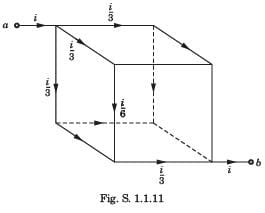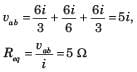QUESTION: 2

### v1 = ?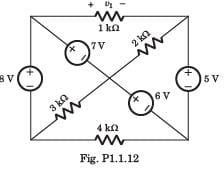Solution:

If we go from +side of 1 kΩ through 7 V, 6 V and 5V,weget v1 = 7+6 + 5 = 8 V

QUESTION: 3

### The voltage vo in fig. P1.1.11 is always equal to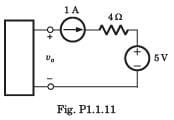Solution:

It is not possible to determine the voltage across 1 A source.

QUESTION: 4

Req = ?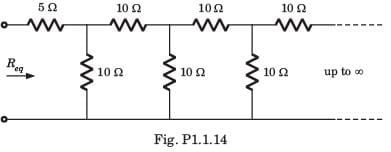Solution: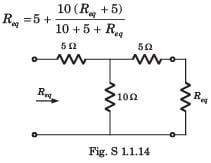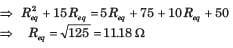QUESTION: 5

In the circuit of fig. P.1.1.31 dependent source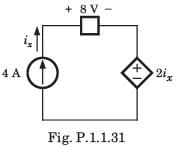Solution: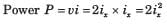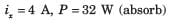QUESTION: 6

A capacitor is charged by a constant current of 2 mA and results in a voltage increase of 12 V in a 10 sec interval. The value of capacitance is

Solution: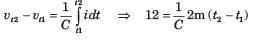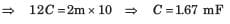QUESTION: 7

The energy required to charge a 10 μF capacitor to 100 V is

Solution: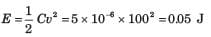QUESTION: 8

The current in a 100 μF capacitor is shown in fig. P.1.1.34. If capacitor is initially uncharged, then the waveform for the voltage across it is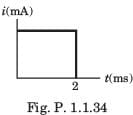Solution: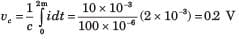This 0.2 V increases linearly from 0 to 0.2 V. Then current is zero. So capacitor hold this voltage.

QUESTION: 9

The voltage across a 100 μF capacitor is shown in fig. P.1.1.35. The waveform for the current in the capacitor is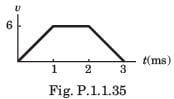Solution: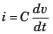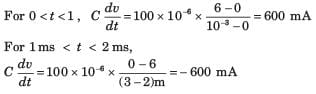QUESTION: 10

The waveform for the current in a 200 μF capacitor is shown in fig. P.1.1.36 The waveform for the capacitor voltage is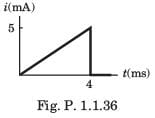Solution:

For 0 ≤ t ≤ 4,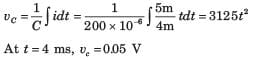It will be parabolic path. at t = 0 t-axis will be tangent.Use Code STAYHOME200 and get INR 200 additional OFF Use Coupon Code